01.图的定义和分类

# 图的定义和分类 #

## 1. 图的定义 #

• 顶点（Vertex）：图中的数据元素通常称为顶点，在下面的示意图中我们使用圆圈来表示顶点。
• 边（Edge）：图中两个数据元素之间的关联关系通常称为边，在下面的示意图中我们使用连接两个顶点之间的线段来表示边。边的形式化定义为：$e = \langle u, v \rangle$，表示从 $u$ 到 $v$ 的一条边，其中 $u$ 称为起始点，$v$ 称为终止点。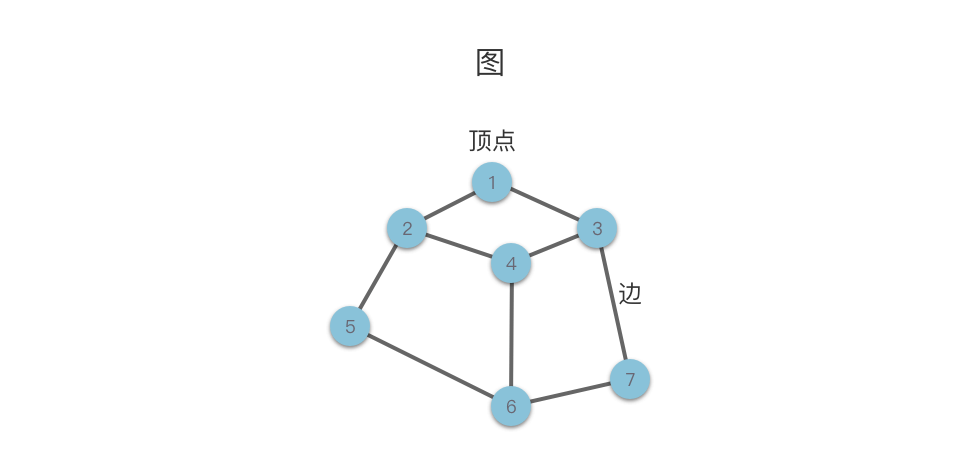• 子图（Sub Graph）：对于图 $G = (V, E)$ 与 $G^{’} = (V^{’}, E^{’})$，如果存在 $V^{’} \subseteq V$，$E^{’} \subseteq E$，则称图 $G^{’}$ 是图 $G$ 的一个子图。在下面的示意图中我们给出了一个图 $G$ 及其一个子图 $G^{’}$。特别的，根据定义，$G$ 也是其自身的子图。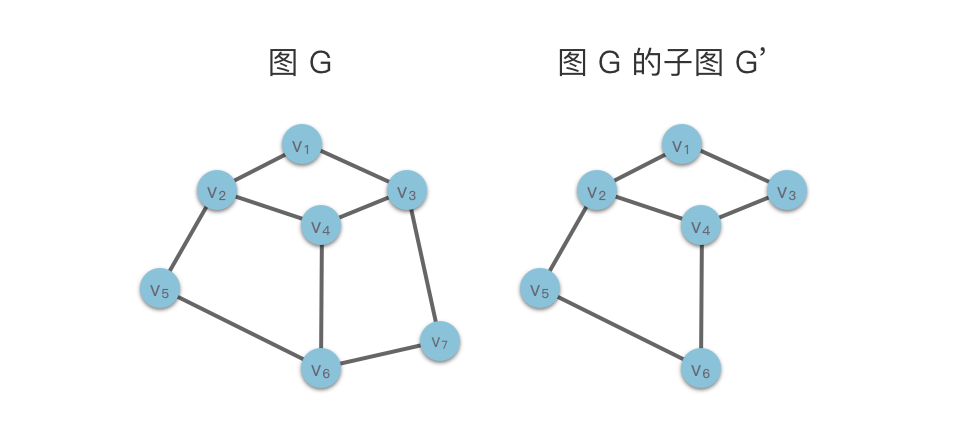## 2. 图的分类 #

### 2.1 无向图和有向图 #

• 无向图（Undirected Graph）：如果图中的每条边都没有指向性，则称为无向图。例如朋友关系图、路线图都是无向图。
• 有向图（Directed Graph）：如果图中的每条边都具有指向性，则称为有向图。例如流程图是有向图。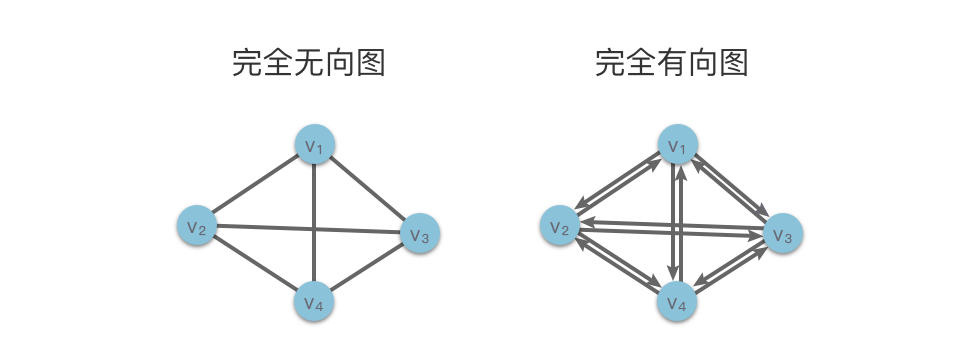• 顶点的度：与该顶点 $v_i$ 相关联的边的条数，记为 $TD(v_i)$。

• 顶点的出度：以该顶点 $v_i$ 为出发点的边的条数，记为 $OD(v_i)$。
• 顶点的入度：以该顶点 $v_i$ 为终止点的边的条数，记为 $ID(v_i)$。
• 有向图中某顶点的度 = 该顶点的出度 + 该顶点的入度，即 $TD(v_i) = OD(v_i) + ID(v_i)$。

### 2.2 环形图和无环图 #

「路径」 是图中的一个重要概念，对于图 $G = (V, E)$，如果存在顶点序列 $v_{i_0}, v_{i_1}, v_{i_2},… , v_{i_m}$，使得 $(v_{i_0}, v_{i_1})，(v_{i_1}, v_{i_2})，…，(v_{i_{m-1}}, v_{i_m}) \in E$（即他们都是图 G 的边，对于有向图则是 $\langle v_{i_0}, v_{i_1} \rangle，\langle v_{i_1}, v_{i_2} \rangle，…，\langle v_{i_{m-1}}, v_{i_m} \rangle \in E$），则称该顶点序列为顶点 $v_{i_0}$ 和顶点 $v_{i_m}$ 之间的一条路径，其中 $v_{i_0}$ 是这条路径的起始点，$v_{i_m}$ 是这条路径的终止点。

• 环（Circle）：如果一条路径的起始点和终止点相同（即 $v_{i_0} = v_{i_m}$ ），则称这条路径为「回路」或者「环」。

• 简单路径：顶点序列中顶点不重复出现的路径称为「简单路径」。

• 环形图（Circular Graph）：如果图中存在至少一条环路，则该图称为「环形图」。
• 无环图（Acyclic Graph）：如果图中不存在环路，则该图称为「无环图」。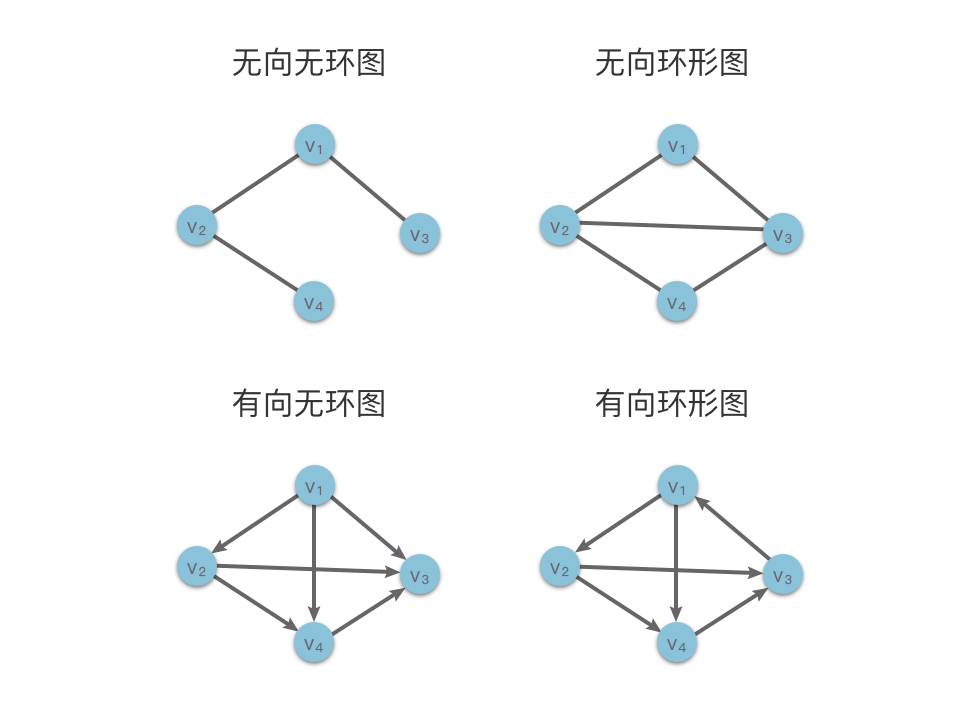### 2.3 连通图和非连通图 #

#### 2.3.1 连通无向图和连通分量 #

• 连通无向图：在无向图中，如果图中任意两个顶点之间都是连通的，则称该图为连通无向图。
• 非连通无向图：在无向图中，如果图中至少存在一对顶点之间不存在任何路径，则该图称为非连通无向图。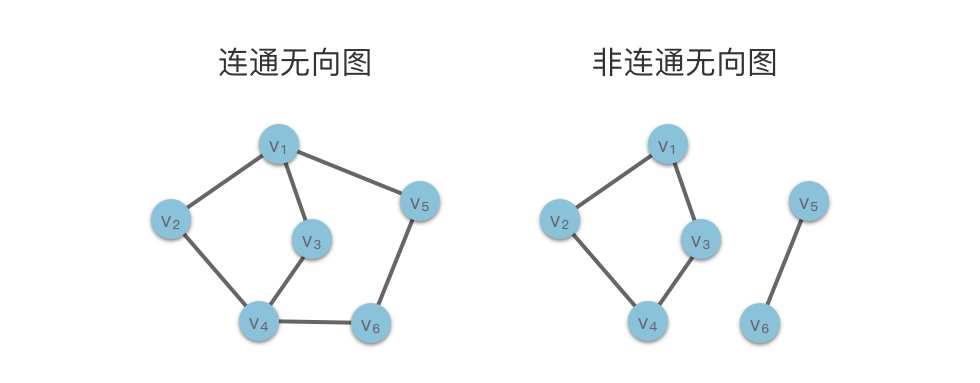• 连通子图：如果无向图的子图是连通无向图，则该子图称为原图的连通子图。
• 连通分量：无向图中的一个极大连通子图（不存在包含它的更大的连通子图）称为该图的连通分量。
• 极⼤连通⼦图：无向图中的一个连通子图，并且不存在包含它的更大的连通子图。

#### 2.3.2 强连通有向图和强连通分量 #

• 强连通有向图：如果图中任意两个顶点 $v_i$ 和 $v_j$，从 $v_i$ 到 $v_j$ 和从 $v_j$ 到 $v_i$ 都有路径，则称该图为强连通有向图。
• 非强连通有向图：如果图中至少存在一对顶点之间不存在任何路径，则该图称为非强连通有向图。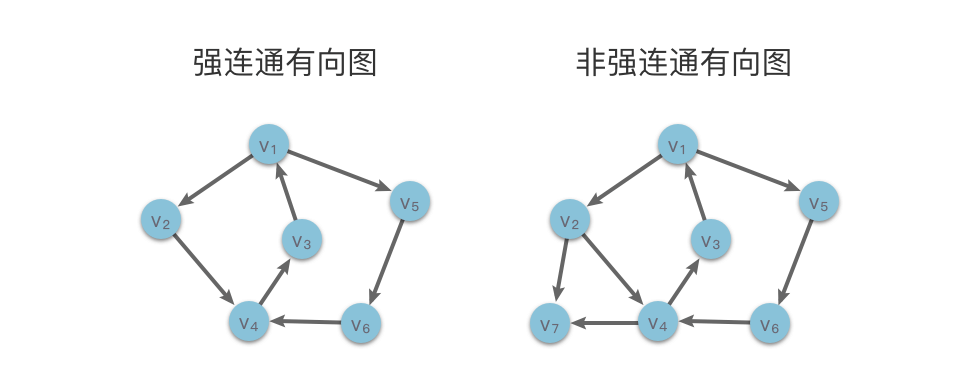• 强连通子图：如果有向图的子图是连通有向图，则该子图称为原图的强连通子图。
• 强连通分量：有向图中的一个极⼤强连通⼦图，称为该图的强连通分量。
• 极⼤强连通⼦图：有向图中的一个强连通子图，并且不存在包含它的更大的强连通子图。

### 2.4 带权图 #

• 带权图：如果图的每条边都被赋以⼀个权值，这种图称为带权图。
• 网络：带权的连通⽆向图称为⽹络。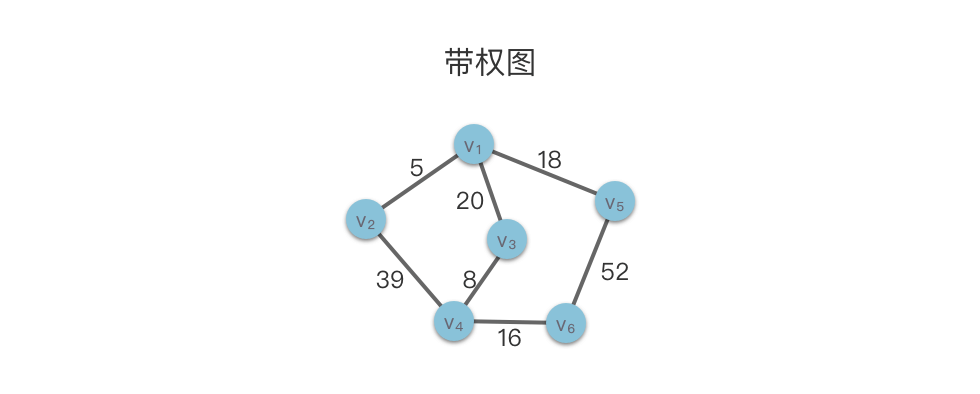### 2.5 稠密图和稀疏图 #

• 稠密图（Dense Graph）：有很多条边或弧（边的条数 e 接近于完全图的边数）的图称为稠密图。
• 稀疏图（Sparse Graph）：有很少条边或弧（边的条数 e 远小于完全图的边数，如 $e < nlog_2n$）的图称为稀疏图。

## 参考资料 #

• 【书籍】ACM-ICPC 程序设计系列 - 图论及应用 - 陈宇 吴昊 主编
• 【书籍】数据结构教程 第 3 版 - 唐发根 著
• 【书籍】大话数据结构 - 程杰 著
• 【书籍】算法训练营 - 陈小玉 著
• 【书籍】Python 数据结构与算法分析 第 2 版 - 布拉德利·米勒 戴维·拉努姆 著
• 【博文】 图的基础知识 | 小浩算法
• 【博文】 链式前向星及其简单应用 | Malash’s Blog